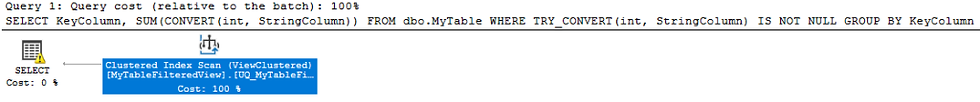top of page
Search

# Filtered Indexes with TRY_CONVERT, ISNUMERIC, and other complex expressions

Who doesn't like Filtered Indexes, am I right? They're pretty great, honestly. If... of course... you're able to actually create them and utilize them in your specific use cases. That... unfortunately, could often become a complicated, if near impossible, task. So, what if our use case is not trivial enough to allow for filtered indexes?## Problem

Assume that you have a table where a string column could potentially hold data represented by other data types such as integers, datetime, and so on.

Let's say that, in order to improve the performance of querying specific data from this table, you want to have a filtered index that would only contain data where, for example, the textual value column is compatible with a certain data type so that it could work well with queries such as this:

```SELECT StringColumn
FROM dbo.MyTable
WHERE ISNUMERIC(StringColumn) = 1```

For example:

```CREATE NONCLUSTERED INDEX IX_ThisWontWork ON dbo.MyTable
(KeyColumn)
INCLUDE(StringColumn)
WHERE ISNUMERIC(StringColumn) = 1```

This will return the following error:

```Msg 10735, Level 15, State 1, Line 1
Incorrect WHERE clause for filtered index 'IX_ThisWontWork' on table 'dbo.MyTable'.```

Indeed, the function ISNUMERIC is not supported within the WHERE clause of a filtered index, and neither many similar functions, such as ISDATE, CONVERT, CAST, TRY_CONVERT, TRY_CAST.

In fact, absolutely no functions of any kind can be used within the WHERE clause of a filtered index. Not even schema-bound user-defined scalar functions.

Unfortunately, as stated in the Microsoft Docs page about Filtered Indexes, the WHERE clause of a filtered index can only support simple comparison operators.

Well, it's not entirely true, as you CAN actually use some functions, but on two conditions:

1. You only send literal values as parameters to them (not columns).

2. That the execution of these functions is on the right side of the equation.

For example, this will work:

```CREATE NONCLUSTERED INDEX IX_LiteralConversion ON dbo.MyTable
(KeyColumn)
WHERE StringColumn = CONVERT(varchar(50), 123)```

But this will not work:

```CREATE NONCLUSTERED INDEX IX_LiteralConversionHuh ON dbo.MyTable
(KeyColumn)
WHERE CONVERT(varchar(50), 123) = StringColumn```

Weird, I know. But this is life. 🙄

## Solution

So, the solution is to... well... not use a filtered index.

Wait, wait, don't walk away in anger!

Because there is an alternative!

Even though the WHERE clause of a filtered index is extremely limited, something that is not as limited is... COMPUTED COLUMNS. And you know what we can do with computed columns? That's right, we can create indexes on top of them!

### Indexed Computed Columns

So, to go back to our original example, what we could do is something like this:

Add a computed column on the table using the ISNUMERIC function:

```ALTER TABLE dbo.MyTable

And then create an index on top of this new column:

```CREATE NONCLUSTERED INDEX IX_Numeric ON dbo.MyTable
(IsNumericComputed, KeyColumn)
INCLUDE(StringColumn)```

(and before you ask - no, we still cannot create a filtered index with the computed column in the filter expression. That's not going to work either, unfortunately)

While this is NOT a filtered index, it can still be used to achieve a similar goal.

For example, this query will successfully utilize the new index and computed column, simply by utilizing an INDEX SEEK:

```SELECT KeyColumn, StringColumn
FROM dbo.MyTable
WHERE IsNumericComputed = 1```

In fact, you could even utilize the "underlying expression" of the computed column instead of explicitly specifying the computed column itself, and the SQL Optimizer will still know to utilize the correct index (this is just part of the magic of computed columns in general):

```SELECT KeyColumn, StringColumn
FROM dbo.MyTable
WHERE CONVERT(bit, ISNUMERIC(StringColumn)) = 1```As you can see in the execution plan, the correct computed column index is used

Needless to say, computed columns have their own set of limitations and restrictions. But they're far more approachable than filtered indexes.

### Indexed Views

One additional drawback to our solution is the fact that we had to modify the structure of our table by adding a computed column. In some systems, this could be a serious issue that could cause code-breaking problems.

Another possible alternative to that is to use Indexed Views. This is, unfortunately, less versatile than a computed column, and comes with its own bundle of problems, restrictions, and limitations.

But, if adding columns to your existing table isn't possible, then this is another alternative that could be worth considering.

For example, if you create this indexed view:

```CREATE VIEW dbo.MyTableFilteredView
WITH SCHEMABINDING
AS
SELECT KeyColumn, SUM(CONVERT(int, StringColumn)) AS TotalValue, COUNT_BIG(*) AS TotalRows
FROM dbo.MyTable
WHERE TRY_CONVERT(int, StringColumn) IS NOT NULL
GROUP BY KeyColumn
GO
CREATE UNIQUE CLUSTERED INDEX UQ_MyTableFilteredView ON dbo.MyTableFilteredView (KeyColumn)
GO```

Then the SQL Optimizer will be able to recognize compatible queries and automatically utilize the indexed view instead of directly accessing the table. For example:

```SELECT KeyColumn, SUM(CONVERT(int, StringColumn))
FROM dbo.MyTable
WHERE TRY_CONVERT(int, StringColumn) IS NOT NULL
GROUP BY KeyColumn```It ain't perfect, and the exact use cases where this is working are rare.

It may be difficult to implement for your specific use case, though, and indexed views have far more impact on DML operations on the table than a computed column.

So, I would definitely strongly recommend finding a way to make computed columns work in your system instead of dealing with indexed views.

## Conclusion

While the solutions we came up with don't necessarily create a filtered sub-set of relevant data as a filtered index should, it still serves as a good performance-enhancing solution since it significantly helps the SQL Optimizer in filtering the relevant data during run-time (by way of an index SEEK on an indexed computed column, or on an indexed view).

Hopefully, this post gave you a good direction to pursue, as an alternative to filtered indexes with complex filter expressions.